# Worksheet on Different Types of Quadrilaterals | Types of Quadrilaterals Worksheets

Solve all problems available on Worksheet on Different Types of Quadrilaterals to get good marks in the exam. You can learn different methods to solve a single problem on the Quadrilateral Practice Worksheets. Check out Free Quadrilateral Worksheets on our website and get a grip on complete Quadrilateral concepts in minutes. Practice using the Types of Quadrilaterals Worksheets available and be familiar with various questions. For your convenience, we even provided step by step solutions to all the problems making it easy for you to understand the problems.

## Solved Problems on Different Types of Quadrilaterals

1. Construct a parallelogram PQRS in which PQ = 5.2 cm, QR = 4.7 cm and PR = 7.6 cm.

Solution:

Steps of Construction:
Given that a parallelogram PQRS in which PQ = 5.2 cm, QR = 4.7 cm and PR = 7.6 cm.
1. Draw a line segment of length 5.2 cm and mark the ends as P and Q.
2. Take the point P as a center and draw an arc by taking the radius 7.6 cm.
3. Next, take point Q as a center and draw an arc by taking the radius 4.7 cm. Mark the point as R where the two arcs cross each other. Join the points Q and R as well as P and R.
Note: A parallelogram is a simple quadrilateral with two pairs of parallel sides. The opposite or facing sides of a parallelogram are of equal length and the opposite angles of a parallelogram are of equal measure.
4. By taking the point P as a center, draw an arc with a radius of 4.7 cm.
5. By taking the point R as a center, draw an arc with a radius of 5.2 cm.
6. Mark the point as S where the two arcs cross each other. Join the points R and S as well as P and S.

PQRS is a required parallelogram.2. Construct a parallelogram PQRS in which PQ = 4.3 cm, PS = 4 cm and QS = 6.8 cm.

Solution:

Steps of Construction:
Given that a parallelogram PQRS in which PQ = 4.3 cm, PS = 4 cm and QS = 6.8 cm.
1. Draw a line segment of length 4.3 cm and mark the ends as P and Q.
2. Take the point P as a center and draw an arc by taking the radius 4 cm.
3. Next, take point Q as a center and draw an arc by taking the radius 6.8 cm. Mark the point as S where the two arcs cross each other. Join the points Q and S as well as P and S.
Note: A parallelogram is a simple quadrilateral with two pairs of parallel sides. The opposite or facing sides of a parallelogram are of equal length and the opposite angles of a parallelogram are of equal measure.
4. By taking the point Q as a center, draw an arc with a radius of 4 cm.
5. By taking the point S as a center, draw an arc with a radius of 4.3 cm.
6. Mark the point as R where the two arcs cross each other. Join the points R and S as well as R and Q.

PQRS is a required parallelogram.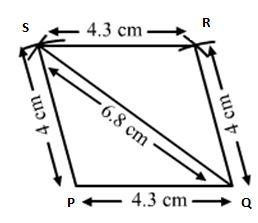3. Construct a parallelogram ABCD in which BC = 6 cm, AB = 4 cm and ∠ABC = 60°.

Solution:

Steps of Construction:
Given that a parallelogram ABCD in which BC = 6 cm, AB = 4 cm and ∠ABC = 60°.
1. Draw a line segment of length 4 cm and mark the ends as A and B.
2. Take point B as a center and make a point by taking 60º using a protector.
3. Next, take point B as a center and draw an arc by taking the radius 6 cm. Mark the point as C where the point and arc cross each other. Join the points C and B.
Note: A parallelogram is a simple quadrilateral with two pairs of parallel sides. The opposite or facing sides of a parallelogram are of equal length and the opposite angles of a parallelogram are of equal measure.
4. By taking the point C as a center, draw an arc with a radius of 4 cm.
5. By taking point A as a center, draw an arc with a radius of 6 cm.
6. Mark the point as D where the two arcs cross each other. Join the points D and C as well as D and A.

ABCD is a required parallelogram.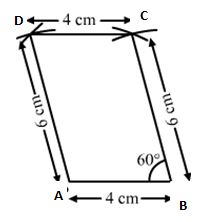4. Construct a parallelogram PQRS in which QR = 5 cm, ∠PQR = 120° and RS = 4.8 cm.

Solution:

Steps of Construction:
Given that a parallelogram PQRS in which QR = 5 cm, ∠PQR = 120° and RS = 4.8 cm.
1. Draw a line segment of length 5 cm and mark the ends as Q and R.
2. Take point R as a center and make a point by taking 120º using a protector.
3. Next, take point R as a center and draw an arc by taking the radius 4.8 cm. Mark the point as S where the point and arc cross each other. Join the points S and R.
Note: A parallelogram is a simple quadrilateral with two pairs of parallel sides. The opposite or facing sides of a parallelogram are of equal length and the opposite angles of a parallelogram are of equal measure.
4. By taking the point S as a center, draw an arc with a radius of 5 cm.
5. By taking point Q as a center, draw an arc with a radius of 4.8 cm.
6. Mark the point as P where the two arcs cross each other. Join the points P and S as well as P and Q.

PQRS is a required parallelogram.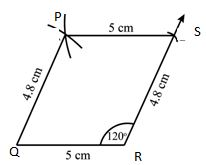5. Construct a parallelogram PQRS, one of whose sides is 4.4 cm and whose diagonals are 5.6 cm and 7 cm. Measure the other side?

Solution:

Steps of Construction:
Given that a parallelogram PQRS, one of whose sides is 4.4 cm and whose diagonals are 5.6 cm and 7 cm.
1. Draw a line segment of length 4.4 cm and mark the ends as P and Q.
2. Make the diagonals half to get the exact vertices of a parallelogram. Take point P as a center and draw an arc by taking the radius 2.8 cm.
3. Next, take point Q as a center and draw an arc by taking the radius 3.5 cm. Mark the point as O where the point and arc cross each other. Join the points P and O, Q and O.
4. Extend the line PO with a radius of 3.5 cm and mark it as R.
5. Extend the line QO with a radius of 2.8 cm and mark it as S.
6. Join the points P and S as well as Q and R, R and S.

PQRS is a required parallelogram.6. Construct a parallelogram PQRS in which PQ = 6.5 cm, PR = 3.4 cm, and the altitude PL from P is 2.5 cm. Draw the altitude from R and measure it?

Solution:

Steps of Construction:
Given that a parallelogram PQRS in which PQ = 6.5 cm, PR = 3.4 cm, and the altitude PL from P is 2.5 cm.
1. Draw a line segment of length 6.5 cm and mark the ends as P and Q.
2. Take point P as a center and draw a perpendicular line PX by taking the radius 2.5 cm and mention that point as L. Draw a parallel line to PQ at a point L.
3. Next, take point P as a center and draw an arc by taking the radius of 3.4 cm. Mark the point as R where the point and arc cross each other. Join the points P and R, Q and R.
4. Take point R as a center and draw an arc by taking the radius of 6.5 cm. Mark the point as S where the point and arc cross each other. Join the points R and S, P and S.

PQRS is a required parallelogram.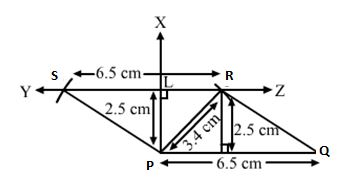7. Construct a parallelogram PQRS, in which diagonal PR = 3.8 cm, diagonal QS = 4.6 cm, and the angle between PR and QS is 60°.

Solution:

Steps of Construction:
Given that a parallelogram PQRS, in which diagonal PR = 3.8 cm, diagonal QS = 4.6 cm, and the angle between PR and QS is 60°.
1. Draw a line segment of length 3.8 cm and mark the ends as P and R.
2. Bisect the line PR and point it as O.
3. Next, take point O and make a point by taking the angle 60° by taking the O as a center.
4. Take point O as a center and draw an arc by taking the radius of 2.3 cm on both sides of O and name them as Q and S. Join the points PQ, QR, RS, SP.

PQRS is a required parallelogram.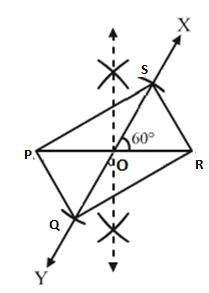8. Construct a rectangle PQRS whose adjacent sides are 11 cm and. 8.5 cm.

Solution:

Steps of Construction:
Given that a rectangle PQRS whose adjacent sides are 11 cm and. 8.5 cm.
1. Draw a line segment of length 11 cm and mark the ends as P and Q.
2. Take point P as a center and make a point by taking 90º using a protector and make the point as E.
Rectangle: All angles of a rectangle are 90º.
3. Next, take point P as a center and draw an arc by taking the radius 8.5 cm. Mark the point as S where the point and arc cross each other. Join the points S and P.
4. Take point Q as a center and draw an arc by taking the radius of 8.5 cm.
5. Also, take point S as a center and draw an arc by taking the radius of 11 cm.
Mark the point as R where the two arcs cross each other. Join the points S and R, Q and R.

PQRS is a required rectangle.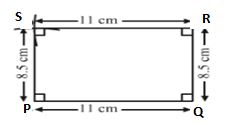9. Construct a square, each of whose sides measures 5.4 cm.

Solution:

Steps of Construction:
Given that a square, each of whose sides measures 5.4 cm.
1. Draw a line segment of length 5.4 cm and mark the ends as P and Q.
2. Take point P as a center and make a point by taking 90º using a protector and make the point as E.
Square: All angles of a square are 90º.
3. Next, take point P as a center and draw an arc by taking the radius 5.4 cm. Mark the point as S where the point and arc cross each other. Join the points S and P.
4. Take point Q as a center and make a point by taking 90º using a protector. Take point Q as a center and draw an arc by taking the radius of 5.4 cm.
5. Also, take point S as a center and draw an arc by taking the radius of 5.4 cm. Mark the point as R where the two arcs cross each other. Join the points S and R, Q and R.

PQRS is a required square.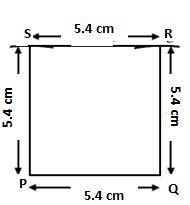10. Construct a square, each of whose diagonals measures 5.6 cm.

Solution:

Steps of Construction:
Given that a square, each of whose diagonals measures 5.6 cm.
1. Draw a line segment of length 5.6 cm and mark the ends as P and R.
2. Bisect the line PR and take half of its radius 2.8 cm. Take point P as a center and draw an arc by taking the radius 2.8 cm.
3. Next, take point R as a center and draw an arc by taking the radius 2.8 cm. Mark the point as S where the point and arc cross each other. Join the points S and P, S and R.
4. Similarly, draw two arcs with 2.8 cms and make the point as Q.
5. Join the points P and Q, S and Q.

PQRS is a required square.11. Construct a rectangle ABCD in which BD = 3.6 cm and diagonal AD = 6 cm. Measure the other side of the rectangle.

Solution:

Steps of Construction:
Given that a rectangle ABCD in which BD = 3.6 cm and diagonal AD = 6 cm.
1. Draw a line segment of length 3.6 cm and mark the ends as B and D.
2. Take point B as a center and make a point by taking 90º using a protector and make the point as E.
Rectangle: All angles of a rectangle are 90º.
3. Next, take point C as a center and draw an arc by taking the radius 6 cm. Mark the point as A where the point and arc cross each other. Join the points A and B, A and D.
4. Similarly, draw two arcs and make the point as C.
5. Join the points A and C, D and C.

ABCD is a required rectangle.12. Construct a rhombus PQRS when the length measures of the diagonals are 8 cm and 6 cm.

Solution:

Steps of Construction:
Given that a rhombus PQRS when the length measures of the diagonals are 8 cm and 6 cm.
1. Draw a line segment of length 8 cm and mark the ends as P and R.
2. Draw perpendicular bisector XY of PR meeting PR at O.
3. Next, From O cut off OS = 1/2 × 6 cm = 3 cm along OX and OQ = 1/2 × 6 cm =3 cm along OY.
4. Join PQ, QR, RS, and SP.

PQRS is a required rhombus.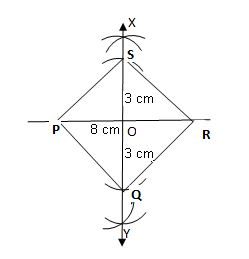13. Construct a rhombus PQRS in which PQ = 4 cm and diagonal PR is 6.5 cm.

Solution:

Steps of Construction:
Given that a rhombus PQRS in which PQ = 4 cm and diagonal PR is 6.5 cm.
1. Draw a line segment of length 4 cm and mark the ends as P and Q.
2. Take the point Q as a center and draw an arc by taking the radius 4 cm.
3. Take the point P as a center and draw an arc by taking the radius 6.5 cm and name it as R.
4. Join PR and QR.
5. Take the point R as a center and draw an arc by taking the radius 4 cm.
6. Take the point P as a center and draw an arc by taking the radius 4 cm. Mark the point as S where the point and arc cross each other. Join the points S and P, S and R.

PQRS is a required rhombus.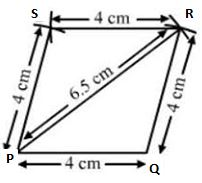14. Draw a rhombus ABCD whose side is 7.2 cm and one angle is 60°.

Solution:

Steps of Construction:
Given that a rhombus ABCD whose side is 7.2 cm and one angle is 60°.
1. Draw a line segment of length 7.2 cm and mark the ends as A and B.
2. Take point A as a center and make a point by taking 60º using a protector.
3. Next, take point A as a center and draw an arc by taking the radius 7.2 cm. Mark the point as D where the point and arc cross each other. Join the points D and A.
4. Take point D as a center and draw an arc by taking the radius 7.2 cm. Take point B as a center and draw an arc by taking the radius of 7.2 cm.
5. Mark the point as C where the two arcs cross each other. Join the points C and B, C and D.

ABCD is a required rhombus.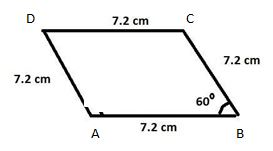15. Construct a trapezium PQRS in which PQ = 6 cm, QR = 4 cm, RS = 3.2 cm, ∠Q = 75° and SR ∥ PQ.

Solution:

Steps of Construction:
Given that a trapezium PQRS in which PQ = 6 cm, QR = 4 cm, RS = 3.2 cm, ∠Q = 75° and SR ∥ PQ.
1. Draw a line segment of length 6 cm and mark the ends as P and Q.
2. Take point Q as a center and make a point by taking 75º using a protector.
3. Next, take point Q as a center and draw an arc by taking the radius 4 cm. Mark the point as R where the point and arc cross each other. Join the points Q and R.
4. RS || PQ, so angle Q + angle R = 180º, angle C = 105º as they are interior angles.
5. Take point R as a center and make a point by taking 105º using a protector.
6. Next, take point R as a center and draw an arc by taking the radius 3.2 cm. Mark the point as S where the point and arc cross each other. Join the points R and S, S and P.

PQRS is a required rhombus.16. Draw a trapezium PQRS in which PQ ∥ SR, PQ = 7 cm, QR = 5 cm, PS = 6.5 cm and ∠Q = 60°.

Solution:

Steps of Construction:
Given that a trapezium PQRS in which PQ ∥ SR, PQ = 7 cm, QR = 5 cm, PS = 6.5 cm and ∠Q = 60°.
1. Draw a line segment of length 7 cm and mark the ends as P and Q.
2. Take point Q as a center and make a point by taking 60º using a protector.
3. Next, take point Q as a center and draw an arc by taking the radius 5 cm. Mark the point as R where the point and arc cross each other. Join the points Q and R.
4. RS || PQ, so angle Q + angle R = 180º, angle C = 120º as they are interior angles.
5. Take point R as a center and make a point by taking 120º using a protector.
6. Next, take point R as a center and draw an arc by taking the radius 6.5 cm. Mark the point as S where the point and arc cross each other. Join the points R and S, S and P.

PQRS is a required rhombus.